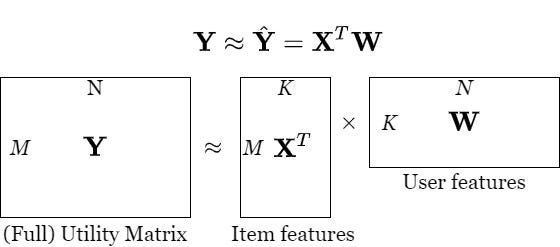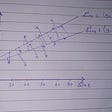# Matrix Factorization Collaborative Filtering — an Explanation

Just a kind note: Since Medium does not support maths equations, many of them in this post were changed to raw representation. In case you dislike reading them (as I do), you can find better content here.

# Introduction

Previously, we introduced collaborative filtering (CF) recommendation system based on the behaviors of users or items in the neighborhood. At this time, another approach to build a CF system is presented — via solving the matrix factorization (MF) or matrix decomposition problem. It is called matrix factorization collaborative filtering (MFCF).

Recall that for content-based recommendation systems, each item is represented by a vector X as an item profile. With this approach, we need to find a vector with coefficient W for each user so that the known ratings of the user on an item is approximately

In that way, utility matrix Y, assuming with all the filled data, approximates to

where M, N are the number of items and users, respectively. Note that with content-based collaborative filtering, X is constructed based on the description data of items and this process is independent of the process of seeking the coefficients of each user. Building item profiles play an important role due to the direct impact on the model’s performance. Furthermore, a drawback of modeling each user is that it does not take advantage of the relationship between users.

Let’s look at the problem from a different angle of view, that is, instead of building item profiles in advance, we build them as feature vectors together with user's models as coefficient vectors. In detail, the variables of the optimization problem here are i) a matrix X of item profiles in which a column is an item, and ii) a matrix W of a user model where each user is represented by a column.

In this way, we approximate the utility matrix Y as the product of two matrices X and W as shown in the following figure. Typically, K is much smaller than M, N and the ranks of both X and W do not exceed K. This method is also known as low-rank matrix factorization illustrated via the following figure.Figure 1: Matrix factorization. The utility matrix Y is the product of two matrices X and W.

Note that:

• The main idea of MF for the recommendation system is the existence of latent features representing the relationship between items and users. While many of you might be curious and try to find out an intuitive explanation for latent features, technically latent features are not something you need to figure out, it’s the job for the system. These hidden features are calculated from observable features and thus are useful to classify items by features (traits) they belong to instead of their classes. Different items expose their latent feature differently via their own vector X. The higher value it is, the more an item exposes against that latent feature. Similarly, each user tends to own some latent properties and represented by elements of the vector w. The value of xw is high if the components of x and w are high (and positive). This means the item has latent properties that the user is interested in, therefore we should suggest that item to the user.
• Why is matrix factorization classified as collaborative filtering? This is based on the fact that the ultimate goal of matrix factorization is to optimize the loss function that will be discussed in the next section. Basically, we need to find X and W as the solutions to the problem, which are dependent on each other in the sense that each X’s column is dependent on all the W’s columns and vice versa.
• In practice, the numbers of items M and of users N are quite large. Finding out a simple model to predict the ratings needs to be done as fast as possible. Neighborhood-based CF (NBCF) does not require too much training, instead, when doing inference, the similarity of the current user to the others needs to be considered. In contrast, by using Matrix Factorization, the learning process can be a bit more complex as it needs to repeatedly optimize one matrix according to the other. MF’s inference step does not require resources for high computing tasks since we only calculate the product of two vectors x and w, with the size K that is much smaller than M and N. MF is thus a more suitable candidate when the problem scale is large.
• One more thing, it requires less memory resource to store matrices x and w comparing to the whole similarity matrix in NBCF. In detail, we need to store K(M+N) elements rather than M² or N² of the similarity matrix.

# Formulate and Optimize the Loss Function

## Approximate existing ratings

As abovementioned, the rating of user \$n\$ on item \$m\$ can be approximated by

Or we can add bias parameters and optimize them. In detail, we have

where b_m and d_n are standalone coefficients corresponding to item m and user n. Let

define the bias vectors for items and users respectively. Like NBCF, these vectors are used to normalize the data where b, d corresponds to item-item CF and user-user CF. But unlike NBCF, they can be tuned to improve the training process. Furthermore, including d and b means we can leverage the advantage of the user-user and item-item CFs into solving the optimization problem.

## Loss Function

Similar to the Content-based Recommendation System, the loss function is constructed based on existing data of the utility matrix Y. The only difference is the absence of bias components and both the matrics Xand W as optimal variables. The additional bias components will be discussed later in this post. The loss function of Matrix Factorization is given as follows:

where r_{mn}=1 if the item m has been rated by the user n, ||.|| is Frobineous norm, i.e. the square root of the sum of the squares of all the elements of the matrix ( like 2-norm of a vector) and s the total number of existing ratings. In the above formula, the first component — data loss is the average of the sum of the squared difference between predicted and actual values, and the second — regularization loss is l_2 regularization to avoid overfitting issue.

`Note: As explained in my previous post about Collaborative Filtering Recommendation System, the ratings are normalized by subtracting the average of a row (or a column) from that row (or that column) elements`

It is quite complicated to optimize X, W, b, d at a time. Instead, we will optimize either (X, b) or (W, d) while fixing the other. This procedure is repeated until the algorithm converges.

## Minimizing Loss Function

By optimizing W while fixing X, we actually go back to the optimization problem used by the Content-based Recommendation System, that is

With the fixed pair (X, b), the optimization problem of the pair (W, d) can be broken into N following sub-problems each of which can be solved by using the gradient descent method.

What we need to do is to calculate the derivative of each function according to w_n and d_n. Since each component of the sum depends on items that are rated by the current user with r_{mn}=1, we can simplify the above formula by constructing a new matrix from the columns of X for the items rated by user n, with the corresponding bias vectors and ratings. Then we have

where 1 is the unit vector (with all elements 1). The partial derivatives of L_1 according to w_n and d_n are

Then we can update w_n, d_n based on the following recursive formula:

Similarly, each column x_m of X as the feature vector of each item, and b_m can be retrieved by optimizing the loss function:

Follow the same steps, we can update x_m and b_m as following:

# Discussion

One advantage of MF for CF is the flexibility with additional constraints that might affect the process of manipulating data or even various applications. One example is related to the normalization process on the ratings. In detail, this process can be included as a part of the loss function. As mentioned in the previous post, the actual ratings are always biased due to the human nature of users and/or items. Some are easy while the others are a bit tougher. Similarly, there are items receiving more ratings than others just because they are already with high ratings and get more ratings from those who have not rated yet. This can be solved with the presence of parameters called biases, which are defined for every user and item and can be tuned together with X and W. Accordingly, the ratings of user n on item m will be approximated to x_mw_n, biases of item m, user n and the average of all the ratings as a constant. Thus we have

The loss function is re-written as follows:

## Nonnegative Matrix Factorization

In general, the data are positive values without normalization. However, if it is not the case, what we need to do is to add a proper value to the utility matrix so as to ensure that all the ratings are positive. From the perspective of MF, there is a widely adopted method with high performance in the Recommendation System, called Nonnegative Matrix Factorization that allows a matrix to be decomposed as a product of positive matrices.

Using MF, users and items are linked via latent features, and how close each user (or item) is to every latent feature is represented by the corresponding element of the feature vector of the user (or item). Apparently, such closeness should be expressed via a non-negative number with 0 indicating the non-relationship between the user (or the item) and the latent feature. And because users and items are typically related to some latent features, the feature vectors should be therefore non-negative with many 0 elements, which can be formulated as non-negative constraints on X and W. That is the idea of the non-negative matrix factorization method.

## Incremental matrix factorization

As mentioned earlier, the predicting time of an MF recommendation system is short whereas the training time is rather long with a large dataset. In fact, the utility matrix keeps changing as new users, items come as well as ratings are inserted or updated, and therefore model’s parameters are required to be updated frequently. As a result, the training process which is already a time-consuming one will need to be performed continuously. This pain can be lessened by using incremental matrix factorization where incremental is explained as minor updates to align to the updated data.

Note that there are various approaches to solve the MF optimization problem apart from gradient descent. To name a few, they are Alternating Least Square, Generalized Low Rank Models, and Singular Value Decomposition. In the next article, I will provide an introduction to the Singular Value Decomposition.

# Acknowledge

I would like to send my big thanks to Vu Huu Tiep for the permission to translate his post.

Originally published at https://techsharing21.com on February 17, 2021.

## More from The Startup

Get smarter at building your thing. Follow to join The Startup’s +8 million monthly readers & +756K followers.

## 7 Reasons Why You Shouldn’t Roll Your Own Computer Vision Pipeline## Linear Regression## Recognizing Handwritten Digits## Implementing Regression Using a Decision Tree and Scikit-Learn## Convolutions: Transposed and Deconvolution## Deep Learning, Convolutional Neural Networks (CNN), Recurrent Neural Networks (RNN), LSTM (Long…## ML | RandomForest Algorithm.## Tuan Nguyen

PhD student, Senior Software Developer

## Graph Analytics for Big Data and its Use-Cases## Graph Data Structure And Its Applications## The Great Upgrade Part Two A## Meet Jennifer Flashman, Director of Analytics at Tinder